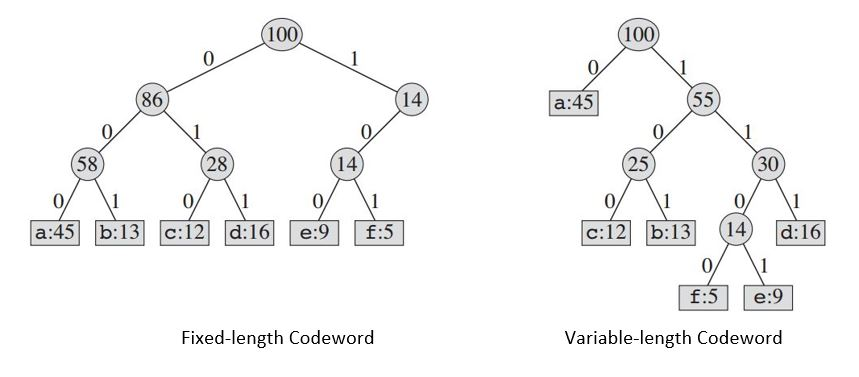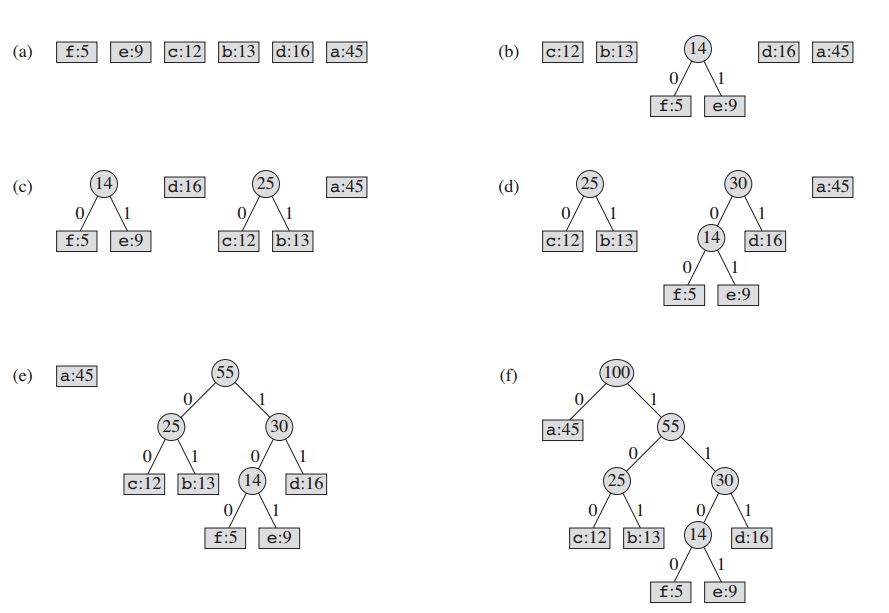# Huffman Coding

suggest change

Huffman code is a particular type of optimal prefix code that is commonly used for lossless data compression. It compresses data very effectively saving from 20% to 90% memory, depending on the characteristics of the data being compressed. We consider the data to be a sequence of characters. Huffman’s greedy algorithm uses a table giving how often each character occurs (i.e., its frequency) to build up an optimal way of representing each character as a binary string. Huffman code was proposed by David A. Huffman in 1951.

Suppose we have a 100,000-character data file that we wish to store compactly. We assume that there are only 6 different characters in that file. The frequency of the characters are given by:

``````+------------------------+-----+-----+-----+-----+-----+-----+
|        Character       |  a  |  b  |  c  |  d  |  e  |  f  |
+------------------------+-----+-----+-----+-----+-----+-----+
|Frequency (in thousands)|  45 |  13 |  12 |  16 |  9  |  5  |
+------------------------+-----+-----+-----+-----+-----+-----+``````

We have many options for how to represent such a file of information. Here, we consider the problem of designing a Binary Character Code in which each character is represented by a unique binary string, which we call a codeword.The constructed tree will provide us with:

``````+------------------------+-----+-----+-----+-----+-----+-----+
|        Character       |  a  |  b  |  c  |  d  |  e  |  f  |
+------------------------+-----+-----+-----+-----+-----+-----+
| Fixed-length Codeword  | 000 | 001 | 010 | 011 | 100 | 101 |
+------------------------+-----+-----+-----+-----+-----+-----+
|Variable-length Codeword|  0  | 101 | 100 | 111 | 1101| 1100|
+------------------------+-----+-----+-----+-----+-----+-----+``````

If we use a fixed-length code, we need three bits to represent 6 characters. This method requires 300,000 bits to code the entire file. Now the question is, can we do better?

A variable-length code can do considerably better than a fixed-length code, by giving frequent characters short codewords and infrequent characters long codewords. This code requires: (45 X 1 + 13 X 3 + 12 X 3 + 16 X 3 + 9 X 4 + 5 X 4) X 1000 = 224000 bits to represent the file, which saves approximately 25% of memory.

One thing to remember, we consider here only codes in which no codeword is also a prefix of some other codeword. These are called prefix codes. For variable-length coding, we code the 3-character file abc as 0.101.100 = 0101100, where “.” denotes the concatenation.

Prefix codes are desirable because they simplify decoding. Since no codeword is a prefix of any other, the codeword that begins an encoded file is unambiguous. We can simply identify the initial codeword, translate it back to the original character, and repeat the decoding process on the remainder of the encoded file. For example, 001011101 parses uniquely as 0.0.101.1101, which decodes to aabe. In short, all the combinations of binary representations are unique. Say for example, if one letter is denoted by 110, no other letter will be denoted by 1101 or 1100. This is because you might face confusion on whether to select 110 or to continue on concatenating the next bit and select that one.

Compression Technique:

The technique works by creating a binary tree of nodes. These can stored in a regular array, the size of which depends on the number of symbols, n. A node can either be a leaf node or an internal node. Initially all nodes are leaf nodes, which contain the symbol itself, its frequency and optionally, a link to its child nodes. As a convention, bit ‘0’ represents left child and bit ‘1’ represents right child. Priority queue is used to store the nodes, which provides the node with lowest frequency when popped. The process is described below:

1. Create a leaf node for each symbol and add it to the priority queue.
2. While there is more than one node in the queue:
``````1. Remove the two nodes of highest priority from the queue.
2. Create a new internal node with these two nodes as children and with frequency equal to the sum of the two nodes' frequency.
3. Add the new node to the queue.``````
1. The remaining node is the root node and the Huffman tree is complete.

For our example:The pseudo-code looks like:

``````Procedure Huffman(C):     // C is the set of n characters and related information
n = C.size
Q = priority_queue()
for i = 1 to n
n = node(C[i])
Q.push(n)
end for
while Q.size() is not equal to 1
Z = new node()
Z.left = x = Q.pop
Z.right = y = Q.pop
Z.frequency = x.frequency + y.frequency
Q.push(Z)
end while
Return Q``````

Although linear-time given sorted input, in general cases of arbitrary input, using this algorithm requires pre-sorting. Thus, since sorting takes O(nlogn) time in general cases, both methods have same complexity.

Since n here is the number of symbols in the alphabet, which is typically very small number (compared to the length of the message to be encoded), time complexity is not very important in the choice of this algorithm.

Decompression Technique:

The process of decompression is simply a matter of translating the stream of prefix codes to individual byte value, usually by traversing the Huffman tree node by node as each bit is read from the input stream. Reaching a leaf node necessarily terminates the search for that particular byte value. The leaf value represents the desired character. Usually the Huffman Tree is constructed using statistically adjusted data on each compression cycle, thus the reconstruction is fairly simple. Otherwise, the information to reconstruct the tree must be sent separately. The pseudo-code:

``````Procedure HuffmanDecompression(root, S):   // root represents the root of Huffman Tree
n := S.length                              // S refers to bit-stream to be decompressed
for i := 1 to n
current = root
while current.left != NULL and current.right != NULL
if S[i] is equal to '0'
current := current.left
else
current := current.right
endif
i := i+1
endwhile
print current.symbol
endfor``````

Greedy Explanation:

Huffman coding looks at the occurrence of each character and stores it as a binary string in an optimal way. The idea is to assign variable-length codes to input input characters, length of the assigned codes are based on the frequencies of corresponding characters. We create a binary tree and operate on it in bottom-up manner so that the least two frequent characters are as far as possible from the root. In this way, the most frequent character gets the smallest code and the least frequent character gets the largest code.

References:

• Introduction to Algorithms - Charles E. Leiserson, Clifford Stein, Ronald Rivest, and Thomas H. Cormen
• Huffman Coding - Wikipedia
• Discrete Mathematics and Its Applications - Kenneth H. Rosen